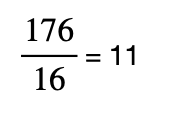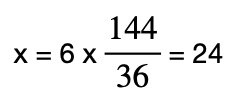# Test: HCF & LCM- 1

## 10 Questions MCQ Test Mathematics for Class 5 | Test: HCF & LCM- 1

Description
Attempt Test: HCF & LCM- 1 | 10 questions in 15 minutes | Mock test for Class 5 preparation | Free important questions MCQ to study Mathematics for Class 5 for Class 5 Exam | Download free PDF with solutions
QUESTION: 1

### Which of these are co-prime (a) 16,18 (b) 11,48 (c) 8,23

Solution:

In number theory, Co-prime numbers are those numbers if the only positive factor that divides them is 1.

Here,

Option A: (16,18) Factors that divide both of them are 1,2.

Option B: (11,48) Factor that divides both of them is 1.

Option C: (8,23) Factor that divides both of them is 1.

In reference to above definition both b and c are co-prime numbers.

QUESTION: 2

### 3 is a factor of 36

Solution:

The factors of 36 are 1, 2, 3, 4, 6, 9, 12, 18, and 36.

Hence, 3 is a factor of 36.

QUESTION: 3

### The LCM of two number is 84. Which one of the following can’t be their HCF.

Solution:

LCM is divisible by HCF.

Hence, 8 cannot be the HCF  because 84 is not divisible by 8.

QUESTION: 4

918573 can be divisible by 3

Solution:

Using divisibility rule:

9+1+8+5+7+3 = 33,

33 is divisible by 3 ( 11×3)

So, 918573 is divisible by 3.

QUESTION: 5

How many prime numbers are there between 1 and 100.

Solution:

The Prime numbers between the numbers 1 to 100 are 2, 3, 5, 7, 11, 13, 17, 19, 23, 29, 31, 37, 41, 43, 47, 53, 59, 61, 67, 71, 73, 79, 83, 89, 97.

Here, we can see that the total count of prime numbers is 25.

QUESTION: 6

1 is prime number

Solution:

No, it is not a prime number.

QUESTION: 7

The factor of a number is less than or equal to that number.

Solution:

A factor of a number is always less than or equal to the given number. Every number except 0 and 1 has at least two factors, 1 and itself.

QUESTION: 8

The LCM of two co- prime number is 176. If one number is 16, find the other number.

Solution:

The product of 2 co-prime numbers is equal to their LCM.

Hence, if one number is 16 other one isQUESTION: 9

HCF of co-prime no. is always

Solution:
• The HCF of two coprime numbers is always 1.
• For example, 5 and 9 are coprime numbers, there, HCF (5, 9) = 1.
QUESTION: 10

The HCF of two numbers is 6 and their LCM is 144. If one of the numbers is 36, the other is

Solution:

Let the other number be x

Product of two numbers = HCF × LCM

x × 36 = 6 × 144Hence, the other number is x = 24Use Code STAYHOME200 and get INR 200 additional OFF Use Coupon Code